• python pandas 数据合并 concat 列名相同，排序相同的3行4df import numpy as np import pandas as pd df1 = pd.DataFrame(np.ones((3,4))*0,columns=['a','b','c','d']) df2 = pd.DataFrame(np.ones((3,4))*1,...
#每天一点点#
python pandas 数据合并 concat
列名相同，排序相同的3行4列df
import numpy as np
import pandas as pd

df1 = pd.DataFrame(np.ones((3,4))*0,columns=['a','b','c','d'])
df2 = pd.DataFrame(np.ones((3,4))*1,columns=['a','b','c','d'])
df3 = pd.DataFrame(np.ones((3,4))*2,columns=['a','b','c','d'])

1：concatenating 方法
1.1 axis=0  纵向相加，axis=1   横向相加
res1 = pd.concat([df1,df2,df3],axis=0) #纵向相加，原数据的什么都不改变，只是纵向相加


输出结果：排序没有变化，可以进一步修改
a    b    c    d
0  0.0  0.0  0.0  0.0
1  0.0  0.0  0.0  0.0
2  0.0  0.0  0.0  0.0
0  1.0  1.0  1.0  1.0
1  1.0  1.0  1.0  1.0
2  1.0  1.0  1.0  1.0
0  2.0  2.0  2.0  2.0
1  2.0  2.0  2.0  2.0
2  2.0  2.0  2.0  2.0
1.2：ignore_index=True  序列号重新排序
res2 = pd.concat([df1,df2,df3],axis=0,ignore_index=True)

输出结果：
a    b    c    d
0  0.0  0.0  0.0  0.0
1  0.0  0.0  0.0  0.0
2  0.0  0.0  0.0  0.0
3  1.0  1.0  1.0  1.0
4  1.0  1.0  1.0  1.0
5  1.0  1.0  1.0  1.0
6  2.0  2.0  2.0  2.0
7  2.0  2.0  2.0  2.0
8  2.0  2.0  2.0  2.0
2：join方法，有 ‘inner’,‘outer’  两种情况
列名不同，排序不同的3行4列df
df1 = pd.DataFrame(np.ones((3,4))*0,columns=['a','b','c','d'],index=[1,2,3])
df2 = pd.DataFrame(np.ones((3,4))*1,columns=['b','c','d','e'],index=[2,3,4])

2.1  默认join是outer，且可省略
res1 = pd.concat([df1,df2],join = 'outer')

输出结果：
a    b    c    d    e
1  0.0  0.0  0.0  0.0  NaN
2  0.0  0.0  0.0  0.0  NaN
3  0.0  0.0  0.0  0.0  NaN
2  NaN  1.0  1.0  1.0  1.0
3  NaN  1.0  1.0  1.0  1.0
4  NaN  1.0  1.0  1.0  1.0
2.2 只join相同列名的内容
res2 = pd.concat([df1,df2],join = 'inner',ignore_index=True) #且重新排序列

输出结果：
b    c    d
0  0.0  0.0  0.0
1  0.0  0.0  0.0
2  0.0  0.0  0.0
3  1.0  1.0  1.0
4  1.0  1.0  1.0
5  1.0  1.0  1.0
3：join_axes 左右横向相加，有点像sql中的left join
3.1 以df1表的序列作为关联
df1 = pd.DataFrame(np.ones((3,4))*0,columns=['a','b','c','d'],index=[1,2,3])
df2 = pd.DataFrame(np.ones((3,4))*1,columns=['b','c','d','e'],index=[2,3,4])
res = pd.concat([df1,df2],axis=1,join_axes=[df1.index])    #以df1表的序列作为关联

输出结果：df2表中没有排序为1的，所以以nan填充
a    b    c    d    b    c    d    e
1  0.0  0.0  0.0  0.0  NaN  NaN  NaN  NaN
2  0.0  0.0  0.0  0.0  1.0  1.0  1.0  1.0
3  0.0  0.0  0.0  0.0  1.0  1.0  1.0  1.0
3.2 直接将两表join
res = pd.concat([df1,df2],axis=1)

输出结果：df2表中没有排序为1的，df4中没有排序为4的，所以以nan填充
a    b    c    d    b    c    d    e
1  0.0  0.0  0.0  0.0  NaN  NaN  NaN  NaN
2  0.0  0.0  0.0  0.0  1.0  1.0  1.0  1.0
3  0.0  0.0  0.0  0.0  1.0  1.0  1.0  1.0
4  NaN  NaN  NaN  NaN  1.0  1.0  1.0  1.0
4：append 方法
df1 = pd.DataFrame(np.ones((3,4))*0,columns=['a','b','c','d'])
df2 = pd.DataFrame(np.ones((3,4))*1,columns=['a','b','c','d'])
df3 = pd.DataFrame(np.ones((3,4))*2,columns=['a','b','c','d'])

res1 = df1.append([df2,df3],ignore_index=True)

输出结果：
a    b    c    d
0  0.0  0.0  0.0  0.0
1  0.0  0.0  0.0  0.0
2  0.0  0.0  0.0  0.0
3  1.0  1.0  1.0  1.0
4  1.0  1.0  1.0  1.0
5  1.0  1.0  1.0  1.0
6  2.0  2.0  2.0  2.0
7  2.0  2.0  2.0  2.0
8  2.0  2.0  2.0  2.0
Series 相结合
df1 = pd.DataFrame(np.ones((3,4))*0,columns=['a','b','c','d'])
s1 = pd.Series([1,2,3,4],index=['a','b','c','d'])
res = df1.append(s1,ignore_index=True)

输出结果：
a    b    c    d
0  0.0  0.0  0.0  0.0
1  0.0  0.0  0.0  0.0
2  0.0  0.0  0.0  0.0
3  1.0  2.0  3.0  4.0


展开全文• 1. 数据合并 前言 ...统计师的Python日记【第6天：数据合并】 前言 根据我的Python学习计划： Numpy → Pandas → 掌握一些数据清洗、规整、合并等功能 → 掌握类似与SQL的聚合等数据...

1. 数据合并
前言

一、横向合并
1. 基本合并语句
2. 键值名不一样的合并
3. “两个数据列名字重复了”的合并

二、纵向堆叠

统计师的Python日记【第6天：数据合并】

前言

根据我的Python学习计划：

Numpy → Pandas → 掌握一些数据清洗、规整、合并等功能 → 掌握类似与SQL的聚合等数据管理功能 → 能够用Python进行统计建模、假设检验等分析技能 → 能用Python打印出100元钱 → 能用Python帮我洗衣服、做饭 → 能用Python给我生小猴子......

前面我把一些基本内容都掌握了，从Python的安装到语句结构、从Numpy/Pandas的数据格式到基本的描述性统计，现在终于要进入一个“应用型”的学习——数据的合并。

其实，我对数据合并很有感情，当年我在某国家医学数据库里实习的时候，就经常用SAS对数据库进行各种合并，以查看受访者在不同数据库中的属性，可以说是使用率非常高的一个技能。

先复习一下几种数据合并方式：左连接（left join）、右连接（right join）、内连接（inner join）、全连接（full join）。

左连接（left join）：以左边的表为基准表，将右边的数据合并过来。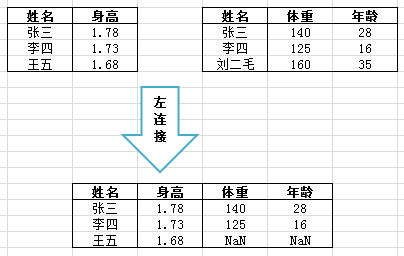右连接（right join）：以右边的表为基准表，将左边的数据合并过来。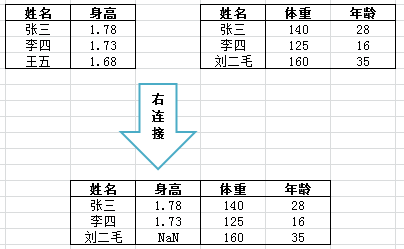内连接（inner join）：左边和右边都出现的数据才进行合并。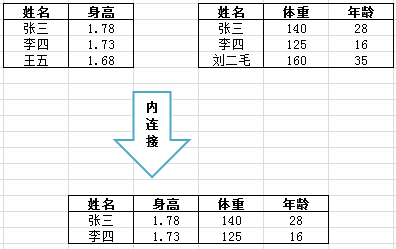全连接（full join）：不管左边还是右边，只要出现的数据都合并过来。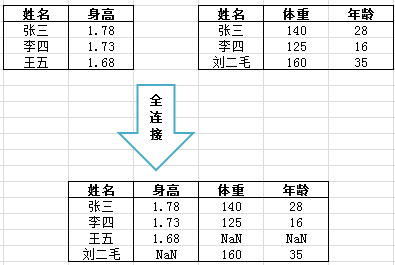以上的几种合并，都是按照姓名来合并的，两个表姓名一样，即将这条数据合并，这个姓名被称为键值，反正叫什么也无所谓，有一个变量被用来作为合并参照就可以了。

OK，今天将学习Python/Pandas的数据合并，合并是基于Pandas这个库，因此首先我们要导入库：import pandas as pd

准备工作完成，开始学习~

一、横向合并

1. 基本合并语句

我有两个数据：

D1 为某洗发店的会员数据，包括会员编号id和会员姓名name。

生成语句为：D1 = pd.DataFrame({'id':[801, 802, 803,804, 805, 806, 807, 808, 809, 810], 'name':['Ansel', 'Wang', 'Jessica', 'Sak','Liu', 'John', 'TT','Walter','Andrew','Song']})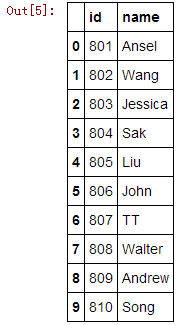D2为该洗发店本月的初值情况，可以看出，本月只有三位会员进行了储值。

生成语句为：D2 = pd.DataFrame({'id':[803, 804, 808,901], 'save': [3000, 500, 1200, 8800]})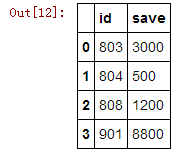现在我想将这两个表合并起来，即 “id-name-save” 的表，键值为id，基本语句为：merge(D1, D2, on='id')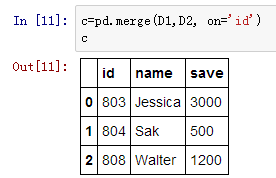哎，我记得合并有左连接、右连接等等，这里我什么也没指定，默认的貌似就是内连接（inner），D1中的801等好几个、D2中的901都没有被合并上，只合并了两个数据中都存在的。

好下面我来左连接，基本语句为：merge(D1, D2, on='id', how='left')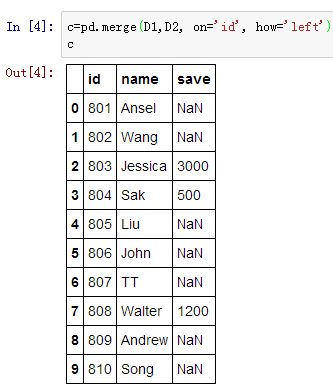D1都被合并进来了，D2的901则没有。

再来一个右连接，基本语句为：merge(D1, D2, on='id', how='right')右边的所有数据都被合并进来了。

全合并的基本语句为：merge(D1, D2, on='id', how='outer')2. 键值名不一样的合并

刚刚的D1和D2，他们都有一个变量id，假如这个键值的名字不一样怎么办？一个叫“id1”、一个叫“id2”。这种情况只要用 left_on= 和 right_on= 分别指定两个键的名字即可，基本语句为：merge(D1, D2, left_on='id1', right_on='id2')我有一个比较变态的问题：如果数据1的键值是变量id，数据2的键值是一个索引，该怎么合并？像这样：也很简单，使用 left_index=True 或 right_index=True，来声明某个数据的索引应该被当做键值，基本语句为：merge(D1, D2, left_on='id', right_index=True)3. 两个数据的列名字重复了

如果两个数据有一样的变量名，那么合并会报错吗？举个例子，现在有803、804、808、901这四位会员3月的储值数据，数据名为D3Month。生成语句为：D3Month= pd.DataFrame({'id':[803, 804, 808, 901], 'save': [3000, 500, 1200, 8800]})

以及四月的储值数据，数据名为D4Month：生成语句为：D4Month= pd.DataFrame({'id':[803, 804, 808, 901], 'save': [0, 1500, 1000, 2000]})

现在想把两个表合并起来，但是两个数据都有save变量，合并之后会报错吗？来看一下吧~没有报错，并且两个save自动打上了后缀，一个是_x，一个是_y，实际上，我们也可以自己加后缀，使用 suffixes=() 选项。比如，我将后缀变为：_3Month和_4Month，基本语句为：merge(D3Month, D4Month, on='id',how='left', suffixes=('_3Month', '_4Month’))二、纵向堆叠

第一部分的内容学习的是将两个数据横向的合并，现在学习纵向合并——也叫做堆叠。比如，我们想象之前的会员数据，被分成了两个部分：

D1：D2：现在咱们再将这两个部分纵向的堆叠起来，注意对这类的堆叠问题，我在以后的日记中尽量不用“合并”这个词（而使用“堆叠”），以便和第一部分的merge区分开来。堆叠的基本语句为：concat([D1,D2])这种情况我在之前的工作中也经常遇到，而且，常常会有这样的需求：堆叠起来的数据，能不能给个标志，标出哪部分来自D1，哪部分来自D2？

肯定可以，用 keys=[ , ] 来标识出来，基本语句为：concat([D1,D2], keys=['D1', 'D2'] )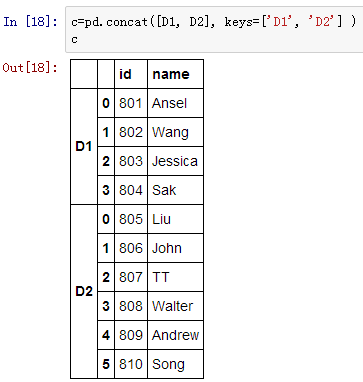当然我们也可以横向堆叠，指定 axis=1，注意喽，虽然是横向，但不是合并（merge），仍然是堆叠，横向堆叠就是粗暴的将两个数据横向堆在一起，请看：仍然可以用 keys=[] 来标识出那边来自D1、哪边来自D2，基本语句为：concat([D1,D2], axis=1, keys=['D1', 'D2'] )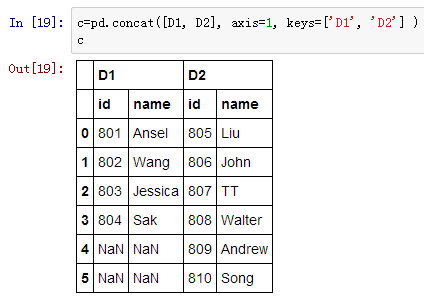转载于:https://www.cnblogs.com/think-and-do/p/6566914.html
展开全文• ## Python数据的合并

千次阅读 2018-06-19 15:45:50
数据合并有两种形式：一种是按照进行合并；一种是按照行进行合并 列合并 merge(data1,data2,how=’left’,out,inner,on=’userid’) 当合并的两个表都没有相同的列名的时候也可以合并，通过制定left_on ,...
数据的合并有两种形式：一种是按照列进行合并；一种是按照行进行合并

列合并
merge(data1,data2,how=’left’,out,inner,on=’userid’)
当合并的两个表都没有相同的列名的时候也可以合并，通过制定left_on ,right_on 来进行合并。
merge(df1,df2,left_on = ,right_on=)
行合并
pandas中使用contact函数进行合并。
contact(df1,df2,df3,axis=1，join=’inner’/’left’)  #axis=1表名使用列进行合并；默认axis=0

展开全文• Numpy 的concatarr1 = np.random.randint(0, 10, (3, 4)) arr2 = np.random.randint(0, 10, (3, 4))np.concatenate(...np.concatenate([arr1, arr2], axis=1)#按照Series 的concat# index 没有重复的情况 ser_obj1 =
Numpy 的concat

arr1 = np.random.randint(0, 10, (3, 4))
arr2 = np.random.randint(0, 10, (3, 4))

np.concatenate([arr1, arr2])
np.concatenate([arr1, arr2], axis=1)#按照列

Series 的concat

# index 没有重复的情况
ser_obj1 = pd.Series(np.random.randint(0, 10, 5), index=range(0,5))
ser_obj2 = pd.Series(np.random.randint(0, 10, 4), index=range(5,9))
ser_obj3 = pd.Series(np.random.randint(0, 10, 3), index=range(9,12))
pd.concat([ser_obj1, ser_obj2, ser_obj3])

# index 有重复的情况
ser_obj1 = pd.Series(np.random.randint(0, 10, 5), index=range(5))
ser_obj2 = pd.Series(np.random.randint(0, 10, 4), index=range(4))
ser_obj3 = pd.Series(np.random.randint(0, 10, 3), index=range(3))
pd.concat([ser_obj1, ser_obj2, ser_obj3], axis=1, join='inner')

DataFrame上的concat

df_obj1 = pd.DataFrame(np.random.randint(0, 10, (3, 2)), index=['a', 'b', 'c'],
columns=['A', 'B'])
df_obj2 = pd.DataFrame(np.random.randint(0, 10, (2, 2)), index=['a', 'b'],
columns=['C', 'D'])

pd.concat([df_obj1, df_obj2])
展开全文• DataFrame中已有pivot()做了数据转换，现要把各合一，作为一 使用pd.concat()完成： ''' #拼接合并，合一 df2=pd.concat(df1.iloc[:,i] for i in range(df1.shape)) #适当修改索引 df2.index=np.arange...DataFrame
• merge 通过键拼接 pandas提供了一个类似于关系数据库的连接(join)操作的方法merage,可以根据一个或多个键将不同DataFrame中的行连接起来 语法如下： merge(left, right, how='inner', on=None, left_on=None, ...
• 按某ID合并两个Excel的数据，首先两个excel中要有相同的一值，才能将这两个表合并起来，合并代码如下： # -*- coding: utf-8 -*- """ Created on Fri Jul 17 16:50:20 2020 """ #按行匹配两个excel中的数据，以...excel
• python提取excel时，首先分析数据构成，确定需求，再按照步骤解决 使用pandas查看数据构成 生成目标数据的dataframe格式 做好目标数据的定位 将列名提取生成列表，循环读取 将文件夹和文件合并为列表做内循环pandas excel
• 合并数据集 轴向合并 数据重塑 移除重复行 参考 合并数据集  pd.merge(left, right, how, on, left_on, right_on, sort)   left 左表 right 右表 how 连接方式 on 连接的列名，...pandas
• Excel Python多表数据合并，跨表取 import openpyxl as xl wb = xl.load_workbook('E:/testE/hahj.xlsx') # 加载excel文件 wb2 = xl.load_workbook('E:/testE/hahj2.xlsx') # 加载excel文件2 wb.create_sheet('...excel
• 方法一： 方法：df['column'] = df['column_A'] + df['column_B'] ...注意：如果某一列是非str类型的数据，那么我们需要用到map(str)将那一列数据类型做转换：df["newColumn"] = df["trans_cd"].map(str) + d...
• 创建数据集 dataDict = {‘a’:[‘one’,‘two’], ‘b’:[[2,3],[1,3,6]], ‘c’:[1,2]} dataDf = pd.DataFrame(dataDict) 实现行转，drop(‘level_2’, axis = 1)：删掉新产生的索引，level_i中的i为groupby...DataFrame
• 解决的问题是提取csv文件和xlsx文件混杂时，文件合并问题。 具体来说，代码是提取指定文本，简单清洗后存入新csv文件。
• Pandas模块是Python用于数据导入及整理的模块，对数据挖掘前期数据的处理工作十分有用 话不多说，先上pandas官方文档： pandas官方文档 数据处理参考文档A 数据处理参考文档B 本次将要实现的需求如下： 1.有一个...
• 首先把所有要提取数据的excel放置到同一个目录文件夹下 导入包： import os '用于提取目录下文件名' import pandas as pd '用于生成data_frame进行结果存储' 提取目录文件夹中所有的文件名： 只需要写到目录文件夹...大数据
• 参考链接：http://pandas.pydata.org/pandas-docs/stable/merging.htmlmerge用于通过一个或多个键将两个数据集的行...在此典型情况下，结果集的行数并没有增加，数则为两个元数据数和减去连接键的数量。 on=N
• 太长不看： pd.concat([p1,p2],axis=1) 使用pands的concat命令pd.concat ...注意两个数据框在中括号内[p1,p2] 数据框1 In : p1 Out: V1 V2 V3 V4 V5 V6 0 1 1 0 0 1 0 1 1 2 0 0 2 0......

# python列数据合并python 订阅